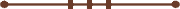####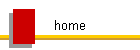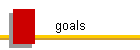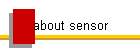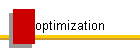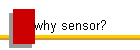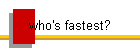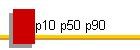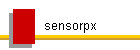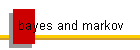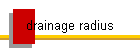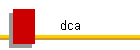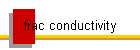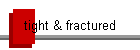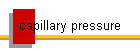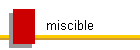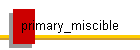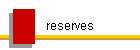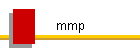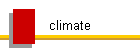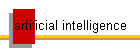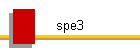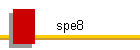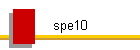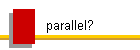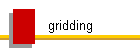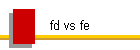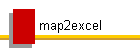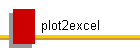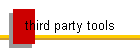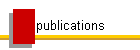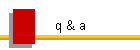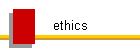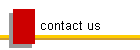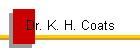#### Modeling of Tight Fractured Reservoirs

Dual porosity and dual permeability (DP) fractured reservoir models were developed decades ago by upscaling orthogonal discrete fracture systems.  They were developed by making the least possible number of simplifying assumptions to obtain a  discrete numerical model that can be practically and robustly solved.  The purposes of DP models are

1. To improve efficiency by combining (upscaling) a group of discrete matrix and surrounding fracture blocks into a reservoir block containing both a single matrix block and a single fracture block.  The upscaling results in an expression for the DP exchange transmissibility Tex between matrix and fracture blocks, as given in the Sensor Manual and originally by Khazemi.  The rate of transfer between each matrix-fracture block pair is independent of fracture permeability, and depends on fracture spacings and matrix permeability and the phase potential gradients representing the difference in capillary and gravity forces.

Tex = 4 (0.0011274) ΔxΔyΔznetm (1-фf) (kx/Lx2+ky/Ly2+kz/Lz2) rb-cp/d-psi

The flow rate of phase j from matrix to fracture is

qj = TexλjΔpj rb/d

where j = w, o, g, for water, oil, and gas, respectively, and

λj = phase j upstream mobility, krjj , 1/cp

krj = phase j upstream relative permeability

Δpj = pjm - pjf, psi

pjm = phase j pressure in matrix

Matrix-fracture transfer can also occur due to diffusion, with similar rate expressions and transfer coefficients (see Sensor Manual).

2. To eliminate the need to conform the grid to any details such as fractures.  A sugarcube approximation specified by gridblock fracture spacing arrays Lx, Ly, Lz allows us to obtain a robust and efficient model solution and to robustly treat the fracture system description as uncertain distributions, the shapes of which are determined by the model, but are strongly guided by experience and expectation, such as extents of the fracture systems that may be detected by microseismic measurements.  This allows the use of a single grid in our attempts to optimize fracture spacings, orientations, distributions, treatments, operating conditions, and production.

If a reservoir has no significant natural fractures, the DP model may not gain any efficiency over the discrete model because the hydraulically fractured blocks are sparse, but it still provides a robust framework for the addition of an empirical geomechanical model representing the effects of fracturing on model parameters.  This allows the fracturing and production processes to be represented in a single model, and eliminates the impossibility of accurate specification of initial conditions in a discrete model after the well has been fractured.  Much more extensive use of available fracture treatment and production data (especially BHP data during fracturing and production) greatly increases our abilities and decreases uncertainty in characterization, history matching, and optimization.

Dual porosity / dual permeability (DP) models have enabled efficient field-scale modeling of naturally fractured reservoirs.  They have historically been validated by comparing DP and discrete model results for immersion tests for one or few-cell systems in which the fractures are flooded with oil or gas and the matrix originally containing hydrocarbon is allowed to equilibrate towards its equilibrium saturations Swe, Sge, from its initial saturations Swi, Sgi.  In conventional fractured or highly heterogeneous systems, the transient time is usually on the order of days to months and is not nearly as important as are the equilibrium matrix saturation distributions Swe and Sge, which (for a water or gas flood) are mainly a function of capillary pressure.  Capillary-gravity equilibrium is achieved between adjacent high-perm (fractures) and low-perm (matrix) regions in the well-swept zones, which are determined by boundary conditions, geology, and sweep efficiency.  This capillary crossflow is described in detail on our Capillary Pressure page with simple conventional examples.

An example comparison of single porosity (SP) and dual porosity (DP) results for an immersion test using Sensor for conventional systems is given at Dual Porosity Immersion Test Examples..  We continue here mainly to focus on issues of applicability to very tight and hydraulically fractured systems.

A common assumption made in modeling naturally fractured reservoirs is that of infinite fracture conductivity, or equivalently infinite fracture permeability.  Pressure drop in the fractures is assumed negligible.  Corresponding "infinite" permeability values are determined as those for which increased values have no effect on results (use of fracture permeabilities that are higher than necessary can cause numerical difficulties, due to difficulty in determining flow directions between small blocks).  If the assumption is valid in a naturally fractured reservoir, it is certainly valid for hydraulic fractures, at least close to the well.

The same principles apply to tight unconventional fractured systems, with matrix permeabilities on the order of nanodarcies and with extremely high capillary pressures due to the much smaller flow paths, or "pore sizes".  In naturally fractured systems, where hydraulic fractures are created, the effects on gridblock Tex values are purely due  to changes in the orthogonal model fracture spacings (the sugarcube DP model gridblocks can be locally orthogonal approximations of input corner-point reservoir gridblocks).  The differences in behavior of conventional and tight systems are mainly due to the fact that the bulk of the matrix in the reservoir will be in transient (changing in saturations towards Swe, Sge but never reaching them) at all times during production.  This places greater demands on the accuracy of the matrix-fracture transfer function Tex in the two-point upscaled DP representation of the discrete matrix-fracture system.

There are many questions and complications regarding the modeling of extremely tight systems, such as modified flow behavior (Klinkenberg effect) and pvt ("bubble point suppression" due to high capillary pressure) in very small pores.  But we will start with the simplest possible assumptions and reservoir and fluid systems in our investigation of the capabilities of reservoir models to optimize well completions, spacings, treatments, operations, and production in very tight systems.  Complexity will be added until we determine the limits of the capabilities of our tools and methods in determining reliable answers to our questions.

##### Gridding Requirements (upscaling)

The first step is to determine the gridding requirements for given simple fracture geometries and distributions in order to obtain an accurate numerical description of flow behavior using either discrete or DP reservoir models.  We will find that these are highly dependent on rock/fluid properties, phase behavior, and initial and boundary conditions, as are the answers to all of our other questions of reservoir behavior.  Some have reported that for real fluids, very fine gridding is required in the matrix adjacent to the fractures in their discrete fracture models.  We will first determine the fine uniform grid solution and then the coarsest sufficient uniform grid for a number of simple problems, and then attempt to find non-uniform grids with sufficient accuracy and better efficiency (fewer number of cells) for some problems of interest.

We start with horizontal 1D flow of a single phase incompressible fluid through 1000 ft of homogeneous slightly compressible matrix into a single fracture on the left end (i=1), with a matrix-fracture area of 10,000 square ft (dy = dz = 100 ft), in a discrete fracture model.   This is a symmetrical pattern element model of a 1/2 of a single planar fracture within semi-infinite matrix.  This case can be solved analytically.  Data are:

single phase incompressible unit mobility fluid (water, Bwi=1, denw=62.4 lb/ft3, visw=1 cp)

arbitrary hydrocarbon pvt

formation compressibility Cf=3.e-6 1/psia, pref=5000 psia

matrix perm Km=30 nd, porosity=.05

i=1 is the fracture block with width delx=.0002 ft, fracture perm = 1D, poros=1 (perm and porosity are irrelevant)

Pinit=5000 psia, well in block 1 (fracture), bhp=14.7 psia, produce for 10 years

df2w.dat, df11w.dat, df21w.dat, df41w.dat, df51w.dat, df101w.dat, and df1001w.dat are 1D discrete fracture models (data files) dfn.dat having n total blocks, the fracture block at i=1 and the n-1 matrix blocks with delx about equal to 1000/(n-1).

Figure 1 indicates that for Km=30 nd, use of uniform matrix blocks as large as 10 ft gives accurate results.  Cumulative water production at 10 years (from df101w.out or df1001w.out) is 101.8 stb.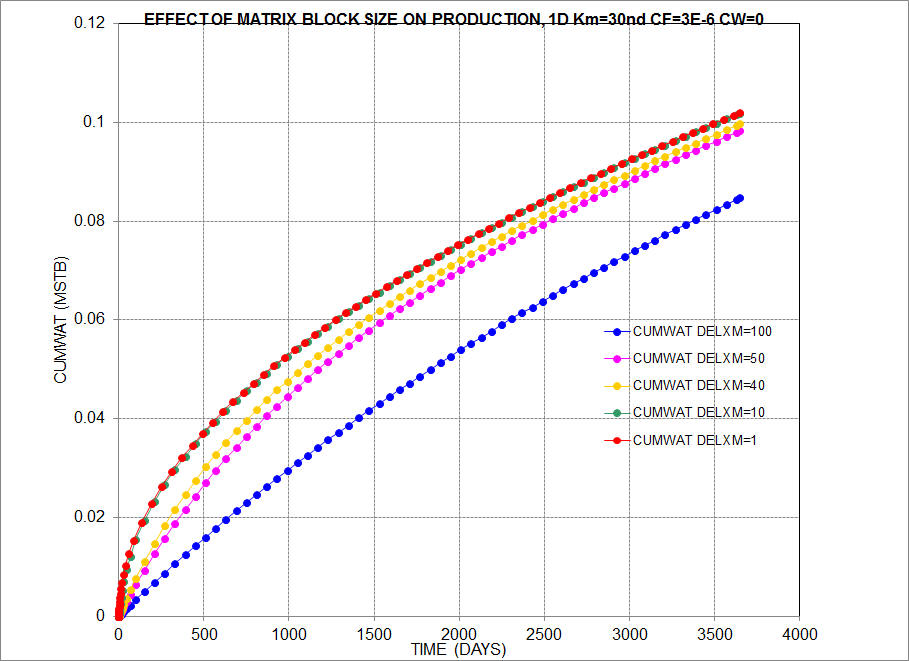Figure 1

Figure 2 shows results for Cf changed from 3.e-6 to 5.e-6 (data files df2wa.dat, df11wa.dat, df21wa.dat, df41wa.dat, df51wa.dat, df101wa.dat, df1001wa.dat).  The maximum block size for good accuracy remains about 10 ft over this range of (small) total compressibility.  Cumulative production at t=10 years increases by about 29% (to 131.3 stb) as a result of the increased formation compressibility.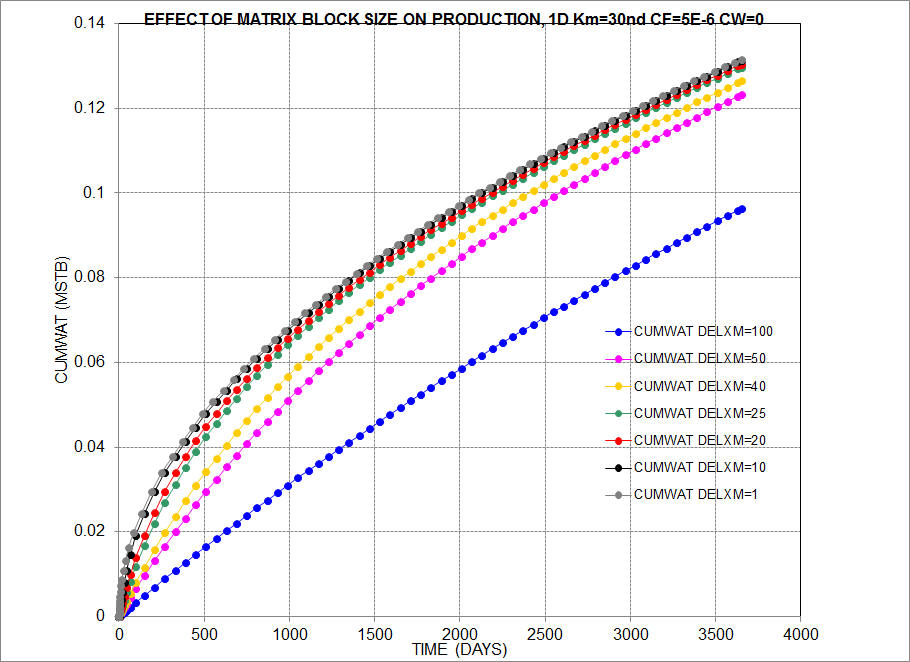Figure 2

Figure 3 shows matrix depletion results for slightly compressible water and formation (Cw=5.e-6 1/psia, Cf=5.e-6 1/psia).  Data files are df2wb.dat df11wb.dat df21wb.dat df41wb.dat, df51wb.dat, df101wb.dat, and df1001wb.dat.  Again, uniform 10 ft matrix blocks give sufficiently accurate results.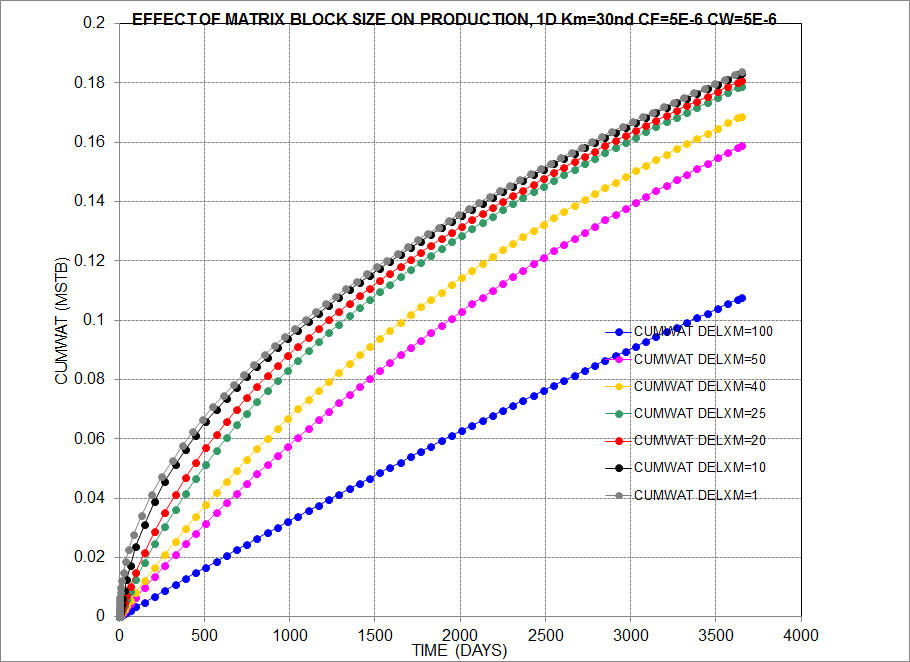Figure 3

Figure 4 shows 1d matrix depletion results for a dry gas rather than water (Swc=.4 in matrix).  Data files are df51wc.dat, df101wc.dat, df501wc.dat, and df1001wc.dat, and df10001wc.dat.  Runs using matrix block sizes of 0.01 ft and 0.1 ft. give virtually identical results.  Use of uniform delx=1 ft matrix blocks gives about 2% error in cumulative production at end of run.  High gas compressibility results in much less propagation of pressure decline into the matrix and in a requirement for much finer gridding than in the all-water case.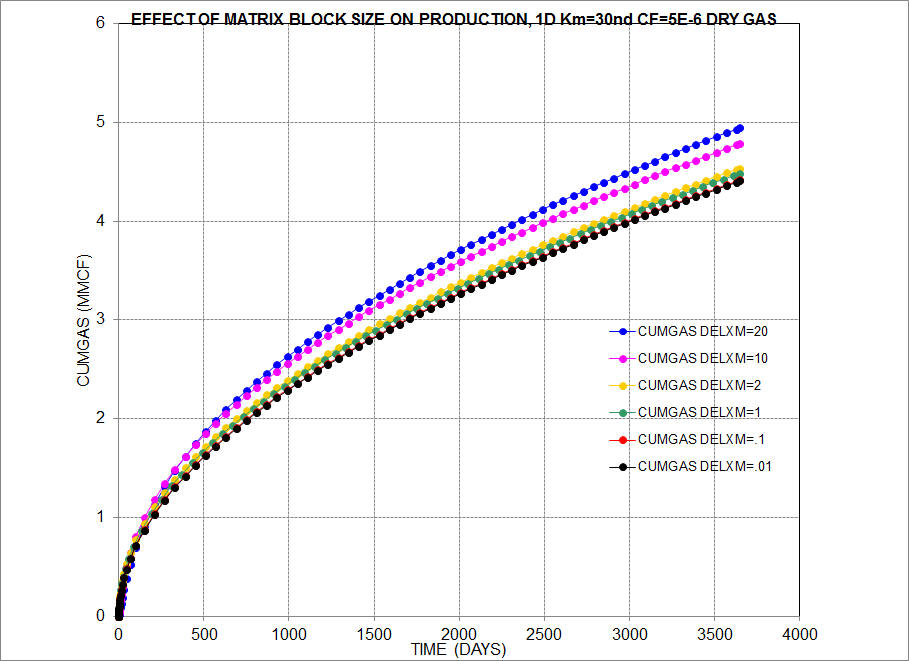Figure 4

Figure 5 shows 1D depletion results for an initially undersaturated oil, using spe1 blackoil pvt data in uniform grids.  Data files are df51d.dat, df101d.dat, df501d.dat, df1001d.dat, df10001d.dat, df100001d.dat.   Again, use of uniform 1 ft grid in the matrix gives about 2% error in cumulative production, and results using 0.1 and 0.01 ft gridblocks are virtually identical.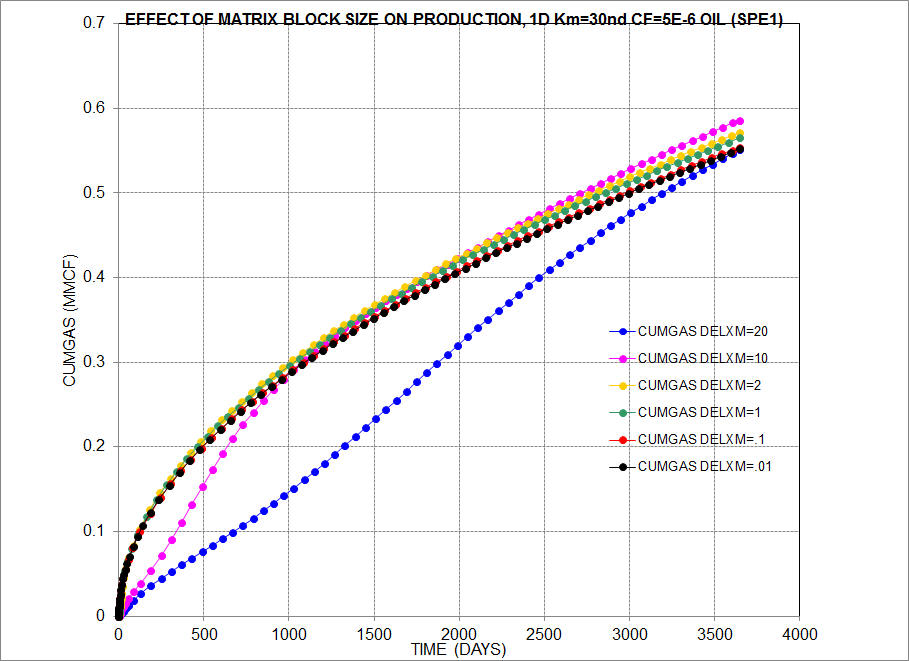Figure 5

Figure 6 shows spe1 depletion results for geometrically spaced grids in the matrix, using a geometric factor g of 1.5 (delx(i+1) = delx(i) * g).  The cases using 20 or more matrix blocks give excellent matches to the fine-grid uniform case (delxm=.01).  Variable grid spacing can maximize performance while preserving accuracy.  It is significantly more accurate than the uniform cell case using 50 times more gridblocks (df1000d.dat), DELXM=1).  The geometric matrix block spacings in the case with 20 matrix blocks are:

0.1504096E+00 0.2256143E+00 0.3384215E+00 0.5076323E+00 0.7614484E+00 0.1142173E+01 0.1713259E+01 0.2569888E+01 0.3854833E+01 0.5782249E+01 0.8673373E+01 0.1301006E+02 0.1951509E+02 0.2927263E+02 0.4390895E+02 0.6586343E+02 0.9879514E+02 0.1481927E+03 0.2222891E+03 0.3334336E+03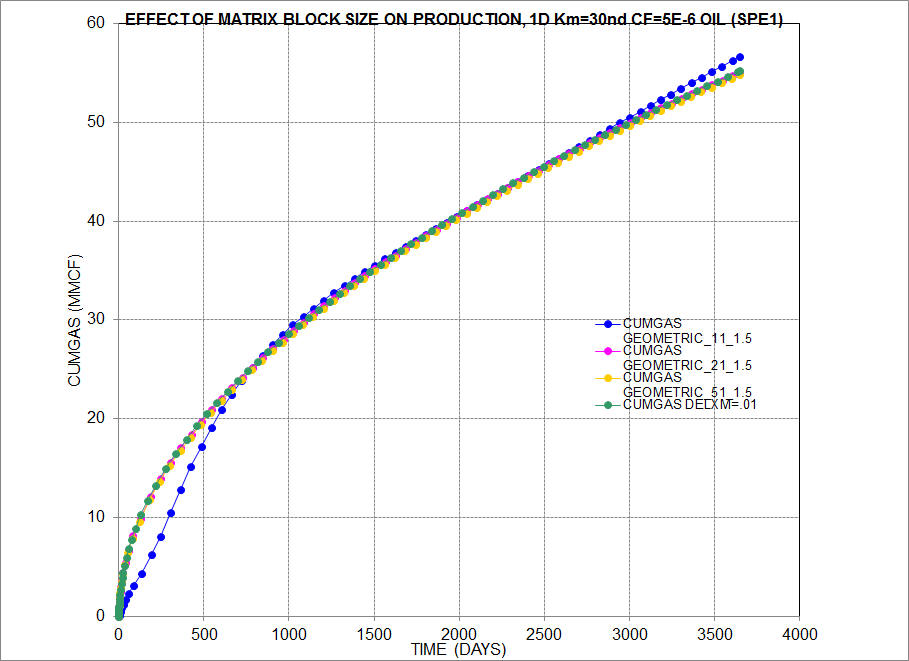Figure 6

The gridding limitations shown so far apply to matrix-fracture flow for discrete fracture single porosity systems.  In dual porosity and dual permeability models, there is no direct flow between a fracture and matrix blocks in adjacent cells (matrix-fracture transfer is instead represented in each cell by the m-f exchange transmissibility Tex).  Dispersion is controlled the same as any other case, by using the largest physical gridblocks for which there is no change in results (over cases using smaller blocks).  There is no lower limit on physical gridblock size in dual systems.  A single fracture can be represented by specifying the minimum fracture spacings which are equal to gridbblock dimensions (for example Lx=dx, indicating 2 fractures, one on each side of the matrix block in the yz plane) and then multiplying the computed Tex value by 0.5.  Cells containing no fracture are treated as single porosity (having fracture porosity or pv equal to zero).

© 2000 - 2022 Coats Engineering, Inc.